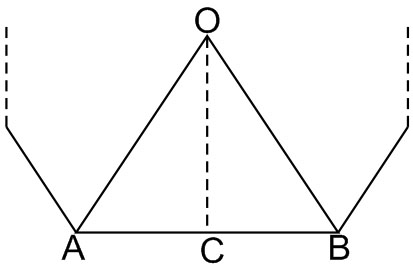#### For a regular polygon, letbe the radii of the inscribed and the circumscribed circles. A false statement among the following is Option 1) there is a regular polygon withOption 2) there is a regular polygon withOption 3) there is a regular polygon withOption 4) there is a regular polygon withAs we learnt in

Relation between Systems of Measurement of Angles -- whereinAngle in DegreesAngle in GradesAngle in Radians

Let O be centre of Polygon, AB be any edge. In radius r = OCThen R = OA = OB,.                       AB = a

Then InIn radiusSimilarly, In{divides (1) by (2)}Option 1)

there is a regular polygon withIncorrect

Option 2)

there is a regular polygon withIncorrect

Option 3)

there is a regular polygon withCorrect

Option 4)

there is a regular polygon withIncorrect

#### Vakul